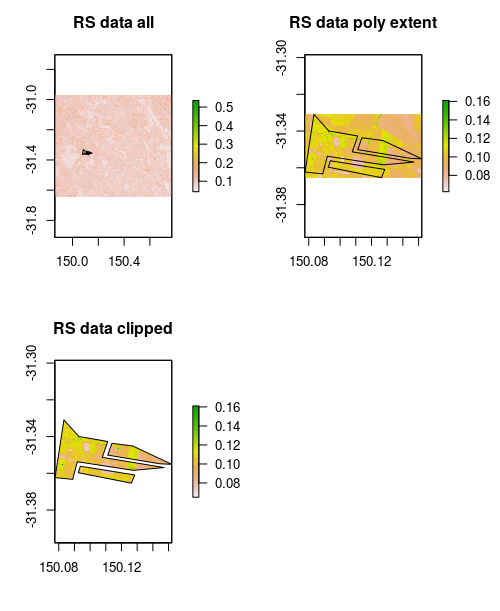## Clipping rasters

In addition to resampling and reprojecting rasters, the other common GIS task needed to harmonise different data source is to clip them too. Usually the clipping is done with the extent, or bounds of another layer usually a polygon, but can be a another raster too. This short piece, shows a workflow for doing this procedure in R where we have a couple of different rasters, and a polygon.

Code is available here

The specific raster data with which to do the exercises is found here

The specific polygon data is found here

You will need to unzip these folders to get at the data.

Initialise the libraries that are needed

library(raster)

library(rgdal)

## rgdal: version: 1.4-8, (SVN revision 845)
##  Geospatial Data Abstraction Library extensions to R successfully loaded
##  Loaded GDAL runtime: GDAL 2.2.3, released 2017/11/20
##  Path to GDAL shared files: /usr/share/gdal/2.2
##  GDAL binary built with GEOS: TRUE
##  Loaded PROJ.4 runtime: Rel. 4.9.3, 15 August 2016, [PJ_VERSION: 493]
##  Path to PROJ.4 shared files: (autodetected)
##  Linking to sp version: 1.3-2

library(sp)


There are three rasters here, but for this exercise we will just use two:

files <- list.files("/~/", pattern = "tif\$", full.names = T)
files

##  "/~/20140509.B3.tif"
##  "/~/elevation.tif"
##  "/~/GR_Th.tif"

# RASTER 1: remote sensing data
rs.raster <- raster(files)
rs.raster

## class      : RasterLayer
## dimensions : 3026, 4037, 12215962  (nrow, ncol, ncell)
## resolution : 0.0002245788, 0.0002245788  (x, y)
## extent     : 149.863, 150.7696, -31.64765, -30.96807  (xmin, xmax, ymin, ymax)
## crs        : +proj=longlat +datum=WGS84 +no_defs +ellps=WGS84 +towgs84=0,0,0
## source     : /~/20140509.B3.tif
## names      : X20140509.B3

# RASTER 2: digital elevation model
elev.raster <- raster(files)
elev.raster

## class      : RasterLayer
## dimensions : 54, 81, 4374  (nrow, ncol, ncell)
## resolution : 100, 100  (x, y)
## extent     : 1510462, 1518562, -3636821, -3631421  (xmin, xmax, ymin, ymax)
## crs        : +proj=lcc +lat_1=-18 +lat_2=-36 +lat_0=0 +lon_0=134 +x_0=0 +y_0=0 +ellps=GRS80 +units=m +no_defs
## source     : /~/elevation.tif
## names      : elevation
## values     : 312.4171, 590.6609  (min, max)


Next we load the polygon data which is called clipper_polygon.shp.

poly.clip <- readOGR("/~/clipper_polygon.shp")

## OGR data source with driver: ESRI Shapefile
## Source: "/~/clipper_polygon.shp", layer: "clipper_polygon"
## with 3 features
## It has 1 fields
## Integer64 fields read as strings:  id


Checking the CRS of each the the data sources, two have the same parameters; rs.raster and poly.clip.

# CRS of data
crs(rs.raster)

## CRS arguments:
##  +proj=longlat +datum=WGS84 +no_defs +ellps=WGS84 +towgs84=0,0,0

crs(elev.raster)

## CRS arguments:
##  +proj=lcc +lat_1=-18 +lat_2=-36 +lat_0=0 +lon_0=134 +x_0=0 +y_0=0
## +ellps=GRS80 +units=m +no_defs

crs(poly.clip)

## CRS arguments:
##  +proj=longlat +datum=WGS84 +no_defs +ellps=WGS84 +towgs84=0,0,0


Below, two workflows are demonstrated.

1. Clip rs.raster with poly.clip

2. Reproject the result from 1 to the same resolution and extent of elev.raster, and also reproject poly.clip to this same CRS. Then clip both rasters simultaneously with the reprojected polygon.

### Clip rs.raster with poly.clip

The basic workflow is to use crop, rasterize, and mask from the raster package in sequential steps to 1) crop the raster data to extent of polygon, 2) rasterise polygon to use as a mask, 3) finalise clipping by applying the mask to the cropped raster.

# crop the rs.raster to extent of polygon
cr <- crop(rs.raster, y = extent(poly.clip), snap = "out")

# rasterise the polygon
fr <- rasterize(x = poly.clip, y = cr)



We can visualise each of the steps in the below figure.Top left plot shows the RS data extent and the relatively smaller polygon situated on it. The top right plot is the cropped RS data, and the bottom left plot is the RS data clipped with the rasterised polygon (mask).

## Clipping multiple rasters simultaneously

First we use projectRaster to make cr.clip from above to have the same CRS, resolution and extent as elev.raster.

## reproject clipped rs.raster to same as elev.raster
reproj.rs.raster <- projectRaster(from = cr.clip, to = elev.raster, method = "bilinear")
reproj.rs.raster

## class      : RasterLayer
## dimensions : 54, 81, 4374  (nrow, ncol, ncell)
## resolution : 100, 100  (x, y)
## extent     : 1510462, 1518562, -3636821, -3631421  (xmin, xmax, ymin, ymax)
## crs        : +proj=lcc +lat_1=-18 +lat_2=-36 +lat_0=0 +lon_0=134 +x_0=0 +y_0=0 +ellps=GRS80 +units=m +no_defs
## source     : memory
## names      : X20140509.B3
## values     : 0.06522314, 0.1324395  (min, max)


Stack the rasters to prepare both for clipping

# stack rasters
stack.raster <- stack(reproj.rs.raster, elev.raster)


Now reproject poly.clip to the same CRS as the stack.raster object created above. Here we are using spTransform from the sp package.

rp.poly.clip <- spTransform(x = poly.clip, CRSobj = crs(stack.raster))


Now clipping proceeds as we did above but this time the target are the two raster in stack.raster.

# crop the rs.raster to extent of polygon
cr <- crop(stack.raster, extent(rp.poly.clip), snap = "out")

# rasterise the polygon
fr <- rasterize(x = rp.poly.clip, y = cr)